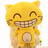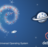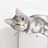V2EX = way to explore
V2EX 是一个关于分享和探索的地方

V2EX  ›  Kotlin

Kotlin 函数与函数式编程

pqpo · 2017-05-25 19:48:59 +08:00 · 4770 次点击

Kotlin 函数

fun main(args: Array) {
var s = sum(1,2)
var m = multi(2,3)
var x = maxOf(3,4)
}

fun sum(a: Int, b: Int): Int {
return a + b
}

fun multi(a: Int, b: Int): Int = a * b

fun maxOf(a: Int, b: Int): Int = if (a > b) a else b

fun main(args: Array) {
isBiggerThan(2)
isBiggerThan(2, 5)
var s = "a".isLetter()
var a = 1 add 2
}

fun isBiggerThan(a: Int, b: Int = 0) {
return a > b
}

//拓展函数
fun String.isLetter(): Boolean {
return matches(Regex("^[a-z|A-Z]\$"))
}

//拓展函数，中缀表达式
infix fun Int.add(x: Int): Int {
return this + x
}

val kv = mapOf("a" to 1, "b" to 2)

public infix fun<A, B> A.to(that: B): Pair<A, B> = Pair(this, that)

Pair 就是 Map 中存的对象，所以你也可以这样创建

val kv = mapOf(Pair("a", 1), Pair("b", 2))

fun main(args: Array) {
val (index, count) = findWhere("abcabcabcabc", 'c')
}

fun findWhere(str: String, findChar: Char): Pair<Int, Int> {
var index = -1
var count = 0
for ((i, v) in str.withIndex()) {
if (v == findChar) {
if (index == -1) {
index = i
}
++count
}
}
return Pair(index, count)
}

for ((k, v) in map) {
print("\$k -> \$v, ")
}

“ Lambda 表达式”(lambda expression)是一个匿名函数，Lambda 表达式基于数学中的λ演算得名，直接对应于其中的 lambda 抽象(lambda abstraction)，是一个匿名函数，即没有函数名的函数。Lambda 表达式可以表示闭包（注意和数学传统意义上的不同）。

Python 中的 lambda 表达式：

C++中的 lambda：

[](int x, int y) -> int{ return x + y; }

Kotlin 中的 lambda:

var add = {x: Int, y: Int -> x + y}

Kotlin 作为一个强类型语言还是比较简洁的。 我们可以这样使用一个 lambda 表达式：

fun main(args: Array) {
val sumLambda = {a: Int, b: Int -> a + b}
sumLambda(1, 2)
}

val numFun: (a: Int, b: Int) -> Int

fun main(args: Array) {
val sumLambda = {a: Int, b: Int -> a + b}
var numFun: (a: Int, b: Int) -> Int
numFun = {a: Int, b: Int -> a + b}
numFun = sumLambda
numFun = ::sum
numFun(1,2)
}

fun sum(a: Int, b: Int): Int {
return a + b
}

using namespace std;

void swap(int &x, int &y);

int main(int arg, char* args[]) {
int x = 10;
int y = 20;

void (*methodPtr)(int &x, int &y);//声明一个函数指针
methodPtr = &swap; //函数指针赋值
methodPtr = swap;//取地址符可省略,效果和上面一致
methodPtr(x, y); //像给函数起了一个别名，可以直接使用（）调用
cout << "x:" << x << " y:" << y << endl; //x:20 y:10
}

void swap(int &x, int &y) {
int tmp = x;
x = y;
y = tmp;
}

//函数参数
fun  doMap(list: List, function: (it: T) -> Any) {
for (item in list) {
function(item)
}
}

val strList = listOf("h" ,"e", "1", "a", "b", "2", " ", "", "c", "5", "7", "F")
doMap(strList, {item ->print("item: \${upperLetter(item)}, ") })

fun upperLetter(item: String): String {
if (item.isLetter()) {
return item.toUpperCase()
}
return item
}

val strList = listOf("h" ,"e", "1", "a", "b", "2", " ", "", "c", "5", "7", "F")
doMap(strList, ::printUpperLetter)

fun printUpperLetter(item: String) {
print("item: \${upperLetter(item)}, ")
}

fun upperLetter(item: String): String {
if (item.isLetter()) {
return item.toUpperCase()
}
return item
}

using namespace std;

void mMap(vector list, void (*fun)(int item));

int main(int arg, char* args[]) {
vector list = {2,3,4,3,2,1,2};
mMap(list, [](int item) -> void { cout << item << endl; });
}

void mMap(vector list, void (*fun)(int item)) {
for(int it : list) {
fun(it);
}
}

fun main(args: Array) {
log { sum(1,2) }
}

fun  log(function: () -> T) {
val result = function()
println("result -> \$result")
}

fun main(args: Array) {
}
//局部函数，函数引用
fun lateAdd(a: Int, b: Int): Function0 {
return a + b
}
}

val funs = mapOf("sum" to ::sum)
val mapFun = funs["sum"]
if (mapFun != null) {
val result = mapFun(1,2)
println("sum result -> \$result")
}

fun sum(a: Int, b: Int): Int {
return a + b
}

fun printUpperLetter(list: List) {
list
.filter (fun(item):Boolean {
return item.isNotEmpty()
})
.filter { item -> item.isNotBlank()}
.filter {
item ->
if (item.isNullOrEmpty()) {
[email protected] false
}
[email protected] item.matches(Regex("^[a-z|A-Z]\$"))
}
.filter { it.isLetter() }
.map(String::toUpperCase)
.sortedBy { it }
.forEach { print("\$it, ") }
println()
}

3 条回复    2017-05-26 13:18:59 +08:001 KeepPro      2017-05-26 01:08:12 +08:00 via Android 感谢楼主科普（安利）2 sagaxu      2017-05-26 08:45:34 +08:003 pqpo   OP   2017-05-26 13:18:59 +08:00 @sagaxu 很生动